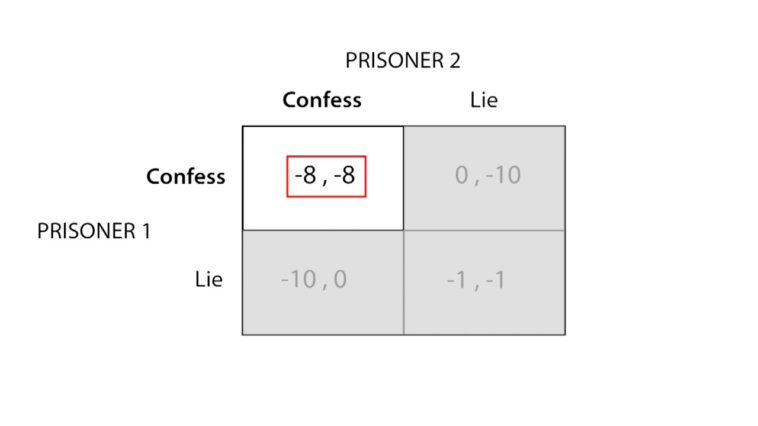in

# Dominant Strategy vs Nash EquilibriumAccording to game theory, the dominant strategy is the optimal move for an individual regardless of how other players act. A Nash equilibrium describes the optimal state of the game where both players make optimal moves but now consider the moves of their opponent.

In contrast with the dominant strategy, it is not necessarily the best move for each player because it may present some conflicts. These conflicts may be due to the numerical disadvantage or due to the inability to see the entire scenario.

## So what is the difference between the dominant strategy and a Nash equilibrium?

Dominant Strategy

The dominant strategy is an action that a player must take, regardless of what other players do. The such strategy is found through an exhaustive examination of all scenarios.

In contrast, a Nash equilibrium is the optimal strategy if a player believes that others will act according to their dominant strategies. It would result in a balance of power and position, hence bringing about an equilibrium in the behavior of the participants.

This is an example of dominant strategy whereby there is a conflict of interest with the other person – the dominant strategy is to win, but if the other players play their own dominant strategy, no one wins.

The Nash equilibrium is a situation where if one of the players makes a change in his strategy then the other person will change his strategy in response to create a new Nash equilibrium.

In this case, both players’ dominant strategy coincides with the other player’s dominant strategy. This is the definition of a Nash equilibrium.

It could be argued that the Nash equilibrium is more relevant to everyday life than the dominant strategy. The fact that we are looking at the same outcomes does not make later a better or worse strategy or game.

## Dominant Strategy vs Nash Equilibrium

The Nash equilibrium is popularly thought of as the best solution to a game whereas the dominant strategy has little value in practical terms. The reason is because the dominant strategy cannot be assessed unless there are no uncertainties.

In contrast, in a game where the players have limited information, a Nash equilibrium is much more useful.

Although the Nash equilibrium is not always optimal, it is almost always better when compared to a dominant strategy. This is because a dominant strategy is static and can’t change when conditions change. In contrast, a Nash equilibrium can change with time thus, improving the overall game.

The best way to understand and remember the difference between dominant strategy and Nash equilibrium is to go over it a number of times.

The fundamental difference between the two strategies comes from

• Dominant Strategy – Defines a solution to the game
• Nash Equilibrium – Defines a stable solution to the game

Another way to look at it is that the Nash equilibrium is a “solution” but dominant strategy defines the optimal “move”.

The conclusion is that a dominant strategy is static and unchanging while a Nash equilibrium is dynamic, changing with time.

Just keep using our visual memory, the best way to memorize content.### Complete Guide to Multi Domestic Strategy